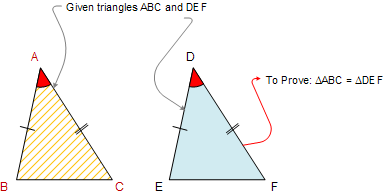Euclid's Elements Book I, Proposition 4: (Side-Angle-Side SAS) If two triangles have two sides equal to two sides respectively, and have the angles contained by the equal sides also equal, then the two triangles are congruent

Let ABC and DEF be two triangles having the two sides AB and AC equal to the two sides DE and DF respectively, and the angle BAC equal to the angle EDF. I say that the base BC also equals the base EF, the triangle ABC equals the triangle DEF, and the remaining angles equal the remaining angles respectively.ConstructionThe Elements: Books I-XIII
 Euclid's Elements is the oldest mathematical and geometric treatise consisting of 13 books written by Euclid in Alexandria c. 300 BC. It is a collection of definitions, postulates, axioms, 467 propositions (theorems and constructions), and mathematical proofs of the propositions.